Select Page

# Ambiguity in Context Free Grammar

There is still no algorithm exists which check ambiguity of grammar. But we use a general approach to find the ambiguity in Context Free grammar.

On the basis of number of derivation trees, ambiguity Grammar is classified into two terms. One is ambiguous grammar and other is Unambiguous grammar.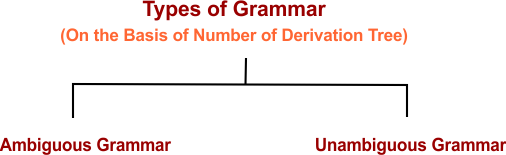# 1. Ambiguous Grammar

A grammar is said to ambiguous grammar if for any string generated by it produces more than one

• Parse tree
• Or syntax tree
• Or leftmost derivation
• Or rightmost derivation

## Examples of Ambiguous Grammar

### Example 01

Check whether the following grammar is ambiguous or not for string w = ab

S → A / B

A → aAb / ab

B → abB / ∈

### Solution

Now we draw more than one parse trees to get string w = ab.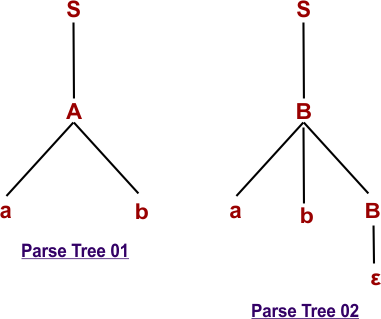As original string (w =ab)  can derived through two different parse trees. So,  the given grammar is ambiguous.

## Example-02

Check whether the following grammar is ambiguous or not for string w = aabbccdd

S → AB / C

A → aAb / ab

B → cBd / cd

D → bDc / bc

### Solution

Now we draw more than one parse trees to get string w = aabbccdd.

##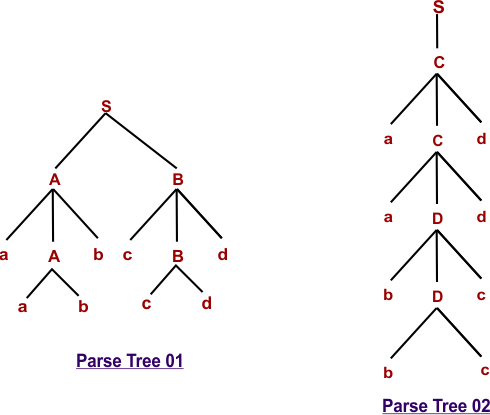As original string (w =aabbccdd)  can derived through two different parse trees. So,  the given grammar is ambiguous.

## 2. Unambiguous Grammar

A grammar is said to unambiguous grammar if for every string generated by it produces exactly the one

• Parse tree
• Or syntax tree
• Or leftmost derivation
• Or rightmost derivation

Note: So, If we try to derive the more than one trees of unambiguous grammar than all trees will be similar

## Examples of Unambiguous Grammar

### Example 01

For string “aab” the following grammar is unambiguous

S → AB

A → Aa | a

B → b

### Solution

Let’s draw leftmost and rightmost derivations of above grammar to get the string “aab”.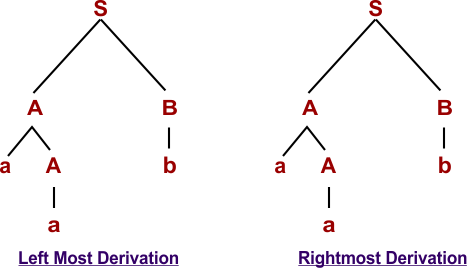Because all parse trees, syntax tress, left or right derivations will be similar for above grammar of string “aab”. So, above grammar is unambiguous.

### Example 02

For string “id+id*id” the following grammar is unambiguous

• E → E + T
• E → T
• T → T * F
• T → F
• F → id

### Solution

Because all parse trees, syntax tress, left or right derivations will be similar for above grammar of string “id+id*id”. As given below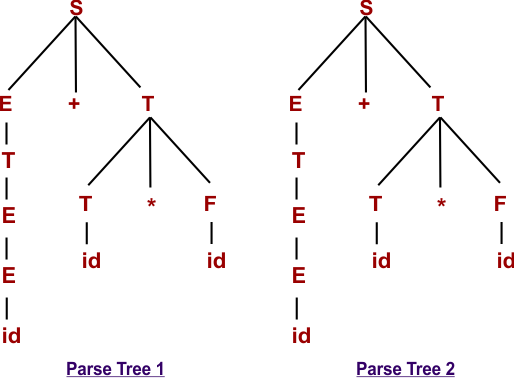## Removal of Ambiguity from CFG

Conversion from ambiguous grammar into an unambiguous grammar is not always possible.

There are some methods which are used for the removal of ambiguity from CFG. Methods are given below

• By fixing the grammar
• By adding the precedence rules
• By using semantics
Help Other’s By Sharing…